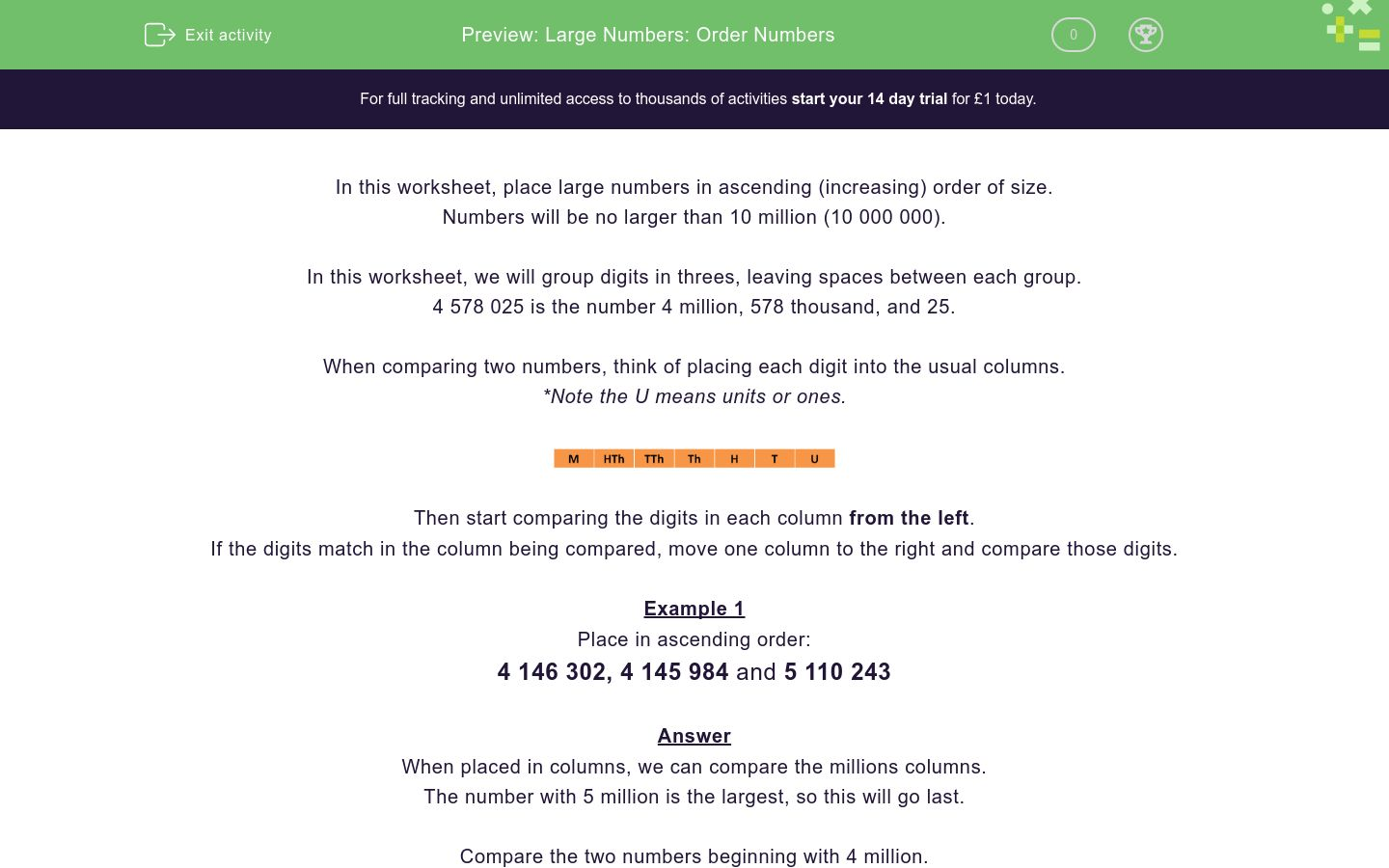# Large Numbers: Order Numbers

In this worksheet, students place numbers up to 10 000 000 in ascending order.Key stage:  KS 2

Curriculum topic:   Number: Number and Place Value

Curriculum subtopic:   Determine Digit Value to 10 000 000

Difficulty level:#### Worksheet Overview

In this worksheet, place large numbers in ascending (increasing) order of size.

Numbers will be no larger than 10 million (10 000 000).

In this worksheet, we will group digits in threes, leaving spaces between each group.

4 578 025 is the number 4 million, 578 thousand, and 25.

When comparing two numbers, think of placing each digit into the usual columns.

*Note the U means units or ones.Then start comparing the digits in each column from the left.

If the digits match in the column being compared, move one column to the right and compare those digits.

Example 1

Place in ascending order:

4 146 302, 4 145 984 and 5 110 243

When placed in columns, we can compare the millions columns.

The number with 5 million is the largest, so this will go last.

Compare the two numbers beginning with 4 million.

The smaller of the two is 4 145 984. (The first three digits match, so compare the thousands column.)

1 (smallest)   4 145 984

2                     4 146 302

3 (largest)      5 110 243

### What is EdPlace?

We're your National Curriculum aligned online education content provider helping each child succeed in English, maths and science from year 1 to GCSE. With an EdPlace account you’ll be able to track and measure progress, helping each child achieve their best. We build confidence and attainment by personalising each child’s learning at a level that suits them.

Get started